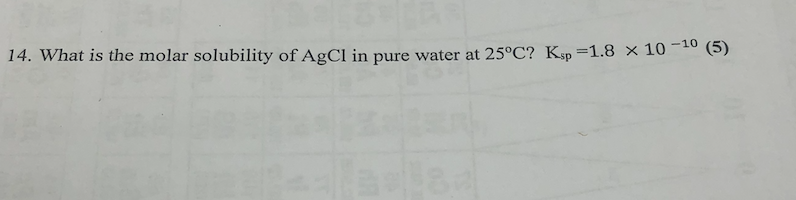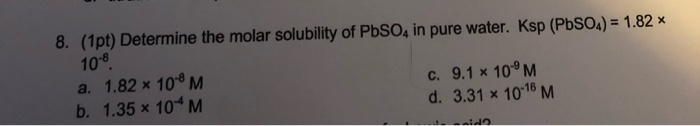# Determine the molar solubility of AgI in pure water. Ksp (AgI)= 8.51 x 10-17

Determine the molar solubility of AgI in pure water.

Ksp (AgI)= 8.51 x 10-17

Ksp=x^2=8.51 x 10^-17

So x=9.22 x 10^-9 M

s^2=8.51*10^-17

So

s=9.225*10^-9 is the molar solubility

let s be the solubility... so s and s dissociates...

=> s^2 = 8.51*10^-17 => s = 9.22*10^-9 M

##### Add Answer of: Determine the molar solubility of AgI in pure water. Ksp (AgI)= 8.51 x 10-17
Similar Homework Help Questions
• ### Determine the molar solubility of PbSO4 in pure water. Ksp(PbSO4)= 1.82 x 10^-8.

Determine the molar solubility of PbSO4 in pure water. Ksp(PbSO4)= 1.82 x 10^-8.

• ### Determine the molar solubility of PbSO4 in pure water. Ksp (PbSO4) = 1.82 times 10-8.

Determine the molar solubility of PbSO4 in pure water. Ksp (PbSO4) = 1.82 times 10-8.

• ### Determine the molar solubility of MgCO3 in pure water. Ksp (MgCO3) = 6.82

Determine the molar solubility of MgCO3 in pure water. Ksp (MgCO3) = 6.82

• ### 14. What is the molar solubility of AgCl in pure water at 25°C? Ksp =1.8 x 1014. What is the molar solubility of AgCl in pure water at 25°C? Ksp =1.8 x 10

• ### Determine the molar solubility of Fe(OH)2 in pure water. Ksp for Fe(OH)2)= 4.87 Ã— 10-17. Please explain your answe...

Determine the molar solubility of Fe(OH)2 in pure water. Ksp for Fe(OH)2)= 4.87 Ã— 10-17. Please explain your answer and I will rate 5 stars! Thanks!

• ### Determine the molar solubility of AgBr in: Ksp (AgBr) = 7.7 x 10^ -13 a. a solution of pure water b. a solution conta...

Determine the molar solubility of AgBr in: Ksp (AgBr) = 7.7 x 10^ -13 a. a solution of pure water b. a solution containing 0.150 M NaBr c. A solution containing 0.25 M NaCL

• ### What is the molar solubility of silver chloride in pure water if its Ksp=1.8*10^-10

What is the molar solubility of silver chloride in pure water if its Ksp=1.8*10^-10

• ### 8. (1pt) Determine the molar solubility of PbSO, in pure water. Ksp (PbSO4) = 1.82 * 10-8 a. 1.82 * 10M C. 9.1 x 10...8. (1pt) Determine the molar solubility of PbSO, in pure water. Ksp (PbSO4) = 1.82 * 10-8 a. 1.82 * 10M C. 9.1 x 10°M b. 1.35 * 10*M d. 3.31 x 10-18 M

• ### Determine the molar solubility for Al(OH)3 in pure water. Ksp for Al(OH)3 = 1.3 x 10-33 2.6 x 10-9 M is the answer,...

Determine the molar solubility for Al(OH)3 in pure water. Ksp for Al(OH)3 = 1.3 x 10-33 2.6 x 10-9 M is the answer, but how? Please explain.

• ### The molar solubility of Ag2S is 1.26 × 10-16 M in pure water. Calculate the Ksp for Ag2S.

The molar solubility of Ag2S is 1.26 × 10-16 M in pure water. Calculate the Ksp for Ag2S.

Need Online Homework Help?